• SNOB 是有限混合模型的 Matlab 实现。 Snob 使用最小消息长度准则来估计混合模型的结构（即子种群的数量；哪个样本属于哪个子种群）并估计所有混合模型参数。 SNOB 允许用户指定所需的子种群数量，但是如果未指定，...matlab
• 针对传统有限混合模型无监督学习算法不能处理参数维数变化的问题,提出了一种基于修正Gibbs 采样的无监督学习算法. 该算法的关键是,在每一次完全采样之后引入分布元的合并和剔除技术,即将利用均值、协方差矩阵差值的2...
• 有限混合模型在应用于数据时非常有用，其中观察来自不同的群体，并且群体隶属关系未知。 模拟数据 首先，我们将模拟一些数据。让我们模拟两个正态分布 - 一个平均值为0，另一个平均值为50，两者的标准差为5。 m1...
原文链接：http://tecdat.cn/?p=6129

介绍

有限混合模型在应用于数据时非常有用，其中观察来自不同的群体，并且群体隶属关系未知。

模拟数据

首先，我们将模拟一些数据。让我们模拟两个正态分布 - 一个平均值为0，另一个平均值为50，两者的标准差为5。

m1 <- 0
m2 <- 50
sd1 <- sd2 <- 5
N1 <- 100
N2 <- 10

a <- rnorm(n=N1, mean=m1, sd=sd1)
b <- rnorm(n=N2, mean=m2, sd=sd2)

现在让我们将数据“混合”在一起......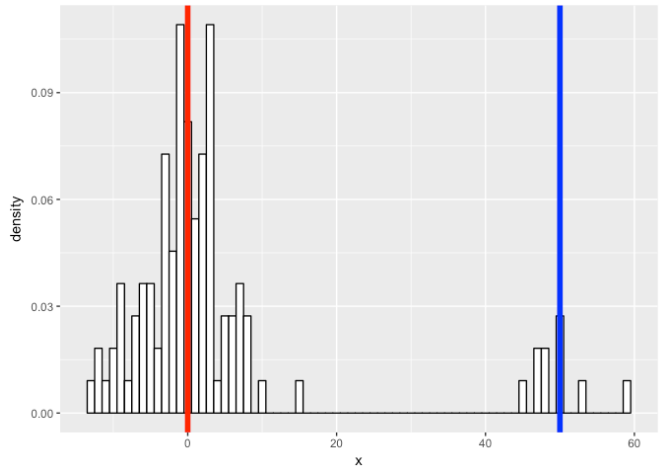print(table(clusters(flexfit), data$class)) ## ## 1 2 ## 1 100 0 ## 2 0 10 参数怎么样？ cat('pred:', c1, '\n') cat('true:', m1, '\n\n') cat('pred:', c1, '\n') cat('true:', sd1, '\n\n') cat('pred:', c2, '\n') cat('true:', m2, '\n\n') cat('pred:', c2, '\n') cat('true:', sd2, '\n\n') ## pred: -0.5613484 ## true: 0 ## ## pred: 4.799484 ## true: 5 ## ## pred: 52.86911 ## true: 50 ## ## pred: 6.89413 ## true: 5 让我们可视化真实数据和我们拟合的混合模型。 ggplot(data) + geom_histogram(aes(x, ..density..), binwidth = 1, colour = "black", fill = "white") + stat_function(geom = "line", fun = plot_mix_comps, args = list(c1, c1, lam/sum(lam)), stat_function(geom = "line", fun = plot_mix_comps, args = list(c2, c2, lam/sum(lam)), colour = "blue", lwd = 1.5) + ylab("Density")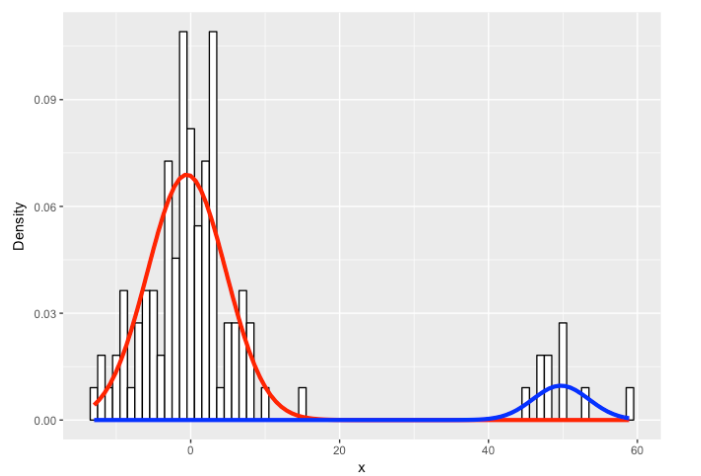看起来我们做得很好！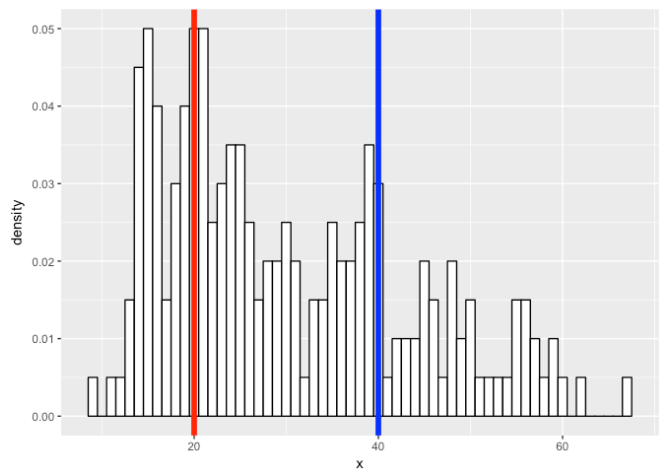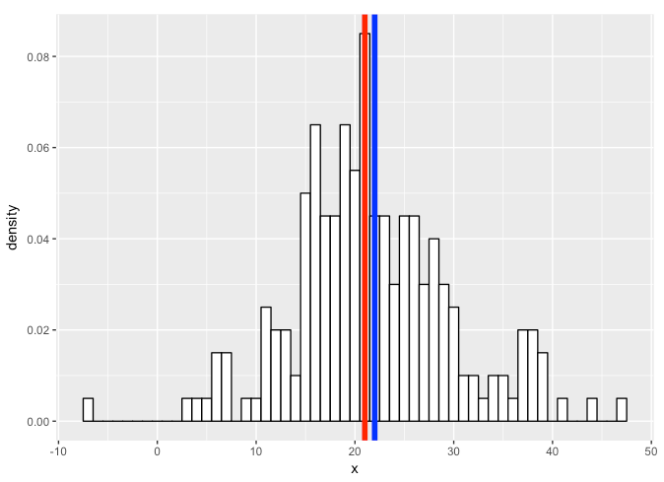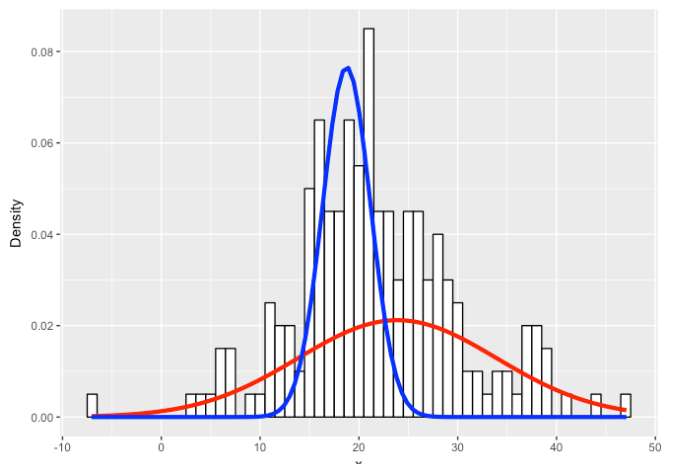例子 现在，让我们考虑一个花瓣宽度为鸢尾花的真实例子。 p <- ggplot(iris, aes(x = Petal.Width)) + geom_histogram(aes(x = Petal.Width, ..density..), binwidth = 0.1, colour = "black", fill = "white") p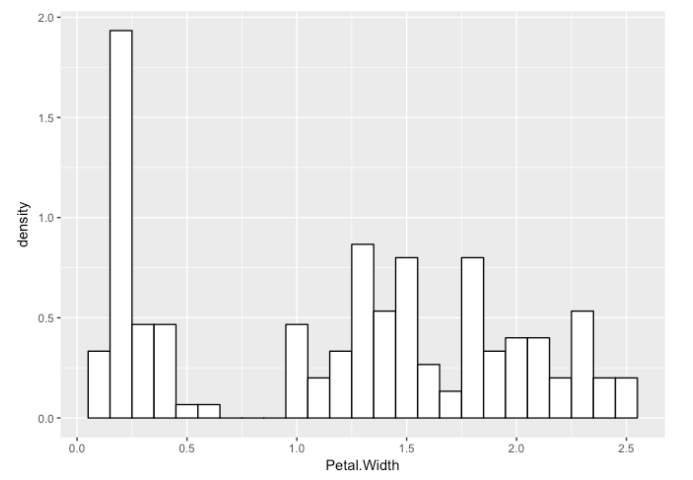flexfit <- flexmix(Petal.Width ~ 1, data = iris, k = 3, model = list(mo1, mo2, mo3)) print(table(clusters(flexfit), iris$Species))
##
##     setosa versicolor virginica
##   1      0          2        46
##   2      0         48         4
##   3     50          0         0

geom_histogram(aes(x = Petal.Width, ..density..), binwidth = 0.1, colour = "black", fill = "white") +
args = list(c1, c1, lam/sum(lam)),
colour = "red", lwd = 1.5) +
stat_function(geom = "line", fun = plot_mix_comps,
args = list(c2, c2, lam/sum(lam)),
stat_function(geom = "line", fun = plot_mix_comps,
args = list(c3, c3, lam/sum(lam)),
colour = "green", lwd = 1.5) +
ylab("Density")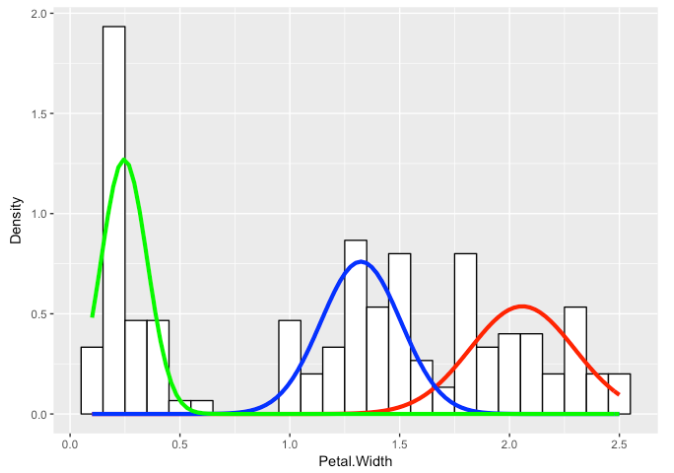即使我们不知道潜在的物种分配，我们也能够对花瓣宽度的基本分布做出某些陈述 。


展开全文R语言
• 既包括传统的方法，如单变量和多变量正态混合的EM算法，也包括反映有限混合模型的一些最新研究的方法。许多算法都是EM算法或基于类似EM的思想，因此本文包括有限混合模型的EM算法的概述。 1.有限混合模型介绍 ...
原文链接：http://tecdat.cn/?p=22609

原文出处：拓端数据部落公众号

摘要

本文提供了一套用于分析各种有限混合模型的方法。既包括传统的方法，如单变量和多变量正态混合的EM算法，也包括反映有限混合模型的一些最新研究的方法。许多算法都是EM算法或基于类似EM的思想，因此本文包括有限混合模型的EM算法的概述。

1.有限混合模型介绍

人群中的个体往往可以被划分为群。然而，即使我们观察到这些个体的特征，我们也可能没有真正观察到这些成员的群体。这项任务在文献中有时被称为 "无监督聚类"，事实上，混合模型一般可以被认为是由被称为 "基于模型的聚类 "的聚类方法的子集组成。

有限混合模型也可用于那些对个体聚类感兴趣的情况之外。首先，有限混合模型给出了整个子群的描述，而不是将个体分配到这些子群中。有时，有限混合模型只是提供了一种充分描述特定分布的手段，例如线性回归模型中存在异常值的残差分布。
无论建模者在采用混合模型时的目标是什么，这些模型的大部分理论都涉及到一个假设，即子群是按照一个特定的参数形式分布的--而这个形式往往是单变量或多变量正态。

最近的研究目标是放宽或修改多变量正态假设，有限混合模型分析的计算技术，其中的成分是回归、多变量数据离散化产生的向量，甚至是完全未指定的分布。

2. 有限混合模型的EM算法

EM算法迭代最大化，而不是观察到的对数似然Lx(θ)，算式为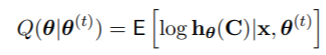1. E步：计算Q(θ|θ(t))
2. M步骤：设定θ(t+1)=argmaxθ∈Φ Q(θ|θ(t))

对于有限混合模型，E步骤不依赖于F的结构，因为缺失数据部分只与Z有关。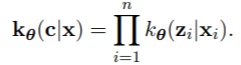Z是离散的，它们的分布是通过贝叶斯定理给出的。M步骤本身可以分成两部分，与λ有关的最大化，它不依赖于F，与φ有关的最大化，它必须为每个模型专门处理（例如，参数化、半参数化或非参数化）。因此，模型的EM算法有以下共同特点。
11. E步。计算成分包含的 "后验 "概率（以数据和θ（t）为条件）。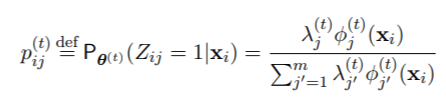对于所有i = 1, . . . ，n和j = 1， . . . 从数值上看，完全按照公式（2）的写法来实现是很危险的，因为在xi离任何一个成分都很远的情况下，所有的φ（t）j 0（xi）值都会导致数值下溢为零，所以可能会出现不确定的形式0/0。因此，许多例程实际上使用的是等价表达式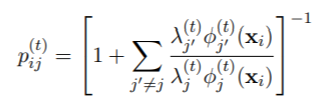或其某种变体。

2.  λ的M步骤。设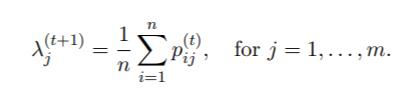2.3. 一个EM算法的例子

作为一个例子，我们考虑对图1中描述的间歇泉喷发间隔时间等待数据进行单变量正态混合分析。这种完全参数化的情况对应于第1节中描述的单变量高斯家族的混合分布，其中（1）中的第j个分量密度φj（x）为正态，均值为μj，方差为σ 2 j。

对于参数(µj , σ2 j )的M步，j = 1, . . 这个EM算法对这种单变量混合分布的M步骤是很简单的，例如可以在McLachlan和Peel（2000）中找到。

mixEM(waiting, lambda = .5)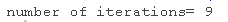上面的代码将拟合一个二成分的混合分布（因为mu是一个长度为2的向量），其中标准偏差被假定为相等（因为sigma是一个标量而不是一个向量）。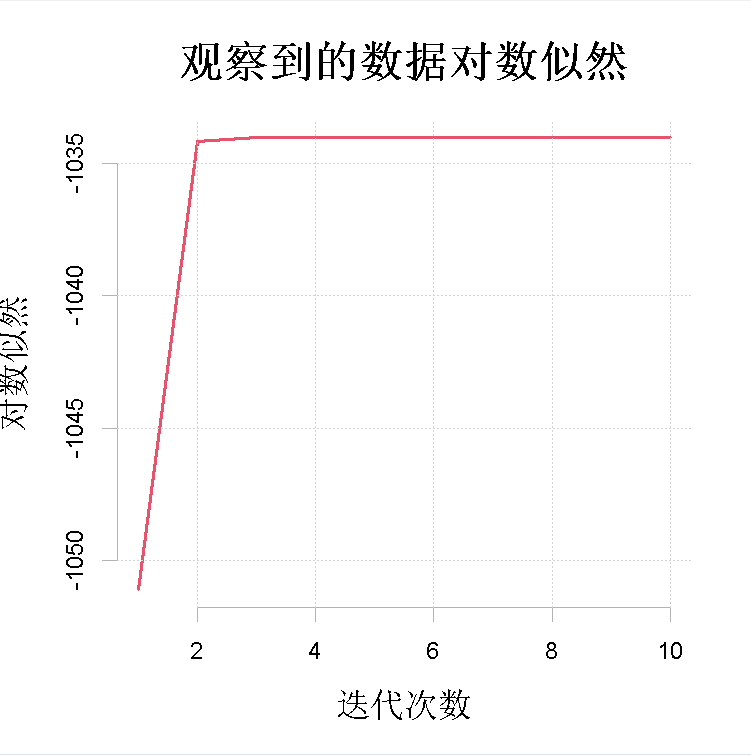图1:对数似然值的序列，Lx(θ (t))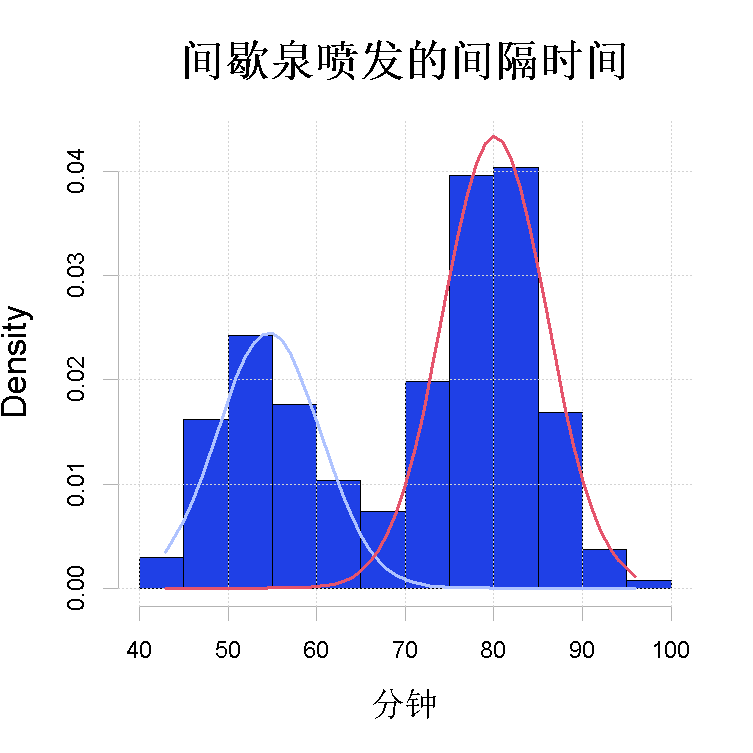图2：用参数化EM算法拟合间歇泉等待数据。拟合的高斯成分。

R> plot(wait1, density = TRUE, cex.axis = 1.4, cex.lab = 1.4, cex.main = 1.8,
+ main2 = "Time between Old Faithful eruptions", xlab2 = "Minutes")

两个图：观察到的对数似然值的序列t 7→Lx(θ (t))和数据的直方图，其中有N(ˆµj , σˆ 2 j)的m(这里m=2)个拟合的高斯分量密度，j=1, . . . ，m，叠加在一起。估计θˆ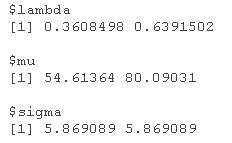另外，使用summary也可以得到同样的输出。

summary(wait1)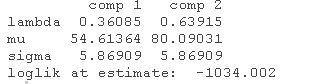3. Cutpoint methods切割点方法

传统上，大多数关于有限混合模型的文献都假设方程（1）的密度函数φj（x）来自一个已知的参数族。然而，一些作者最近考虑了这样的问题：除了确保模型中参数的可识别性所需的一些条件外，φj（x）是不指定的。我们使用Elmore等人（2004）的切割点方法。
我们参考Elmore等人从-63开始，一直到63大约以10.5的间隔采用切点。然后从原始数据中创建一个多指标数据集，如下所示。

R> cutpts <- 10.5*(-6:6)
R> mult(data, cuts = cutpts)


一旦创建了多指标数据，我们可以应用EM算法估计多指标参数。最后，计算并绘制出方程的估计分布函数。图3给出了3分量和4分量解决方案的图表；这些图表与Elmore等人（2004）的图1和图2中的相应图表非常相似。


R> plot(data, posterior, lwd = 2,
+ main = "三分量解")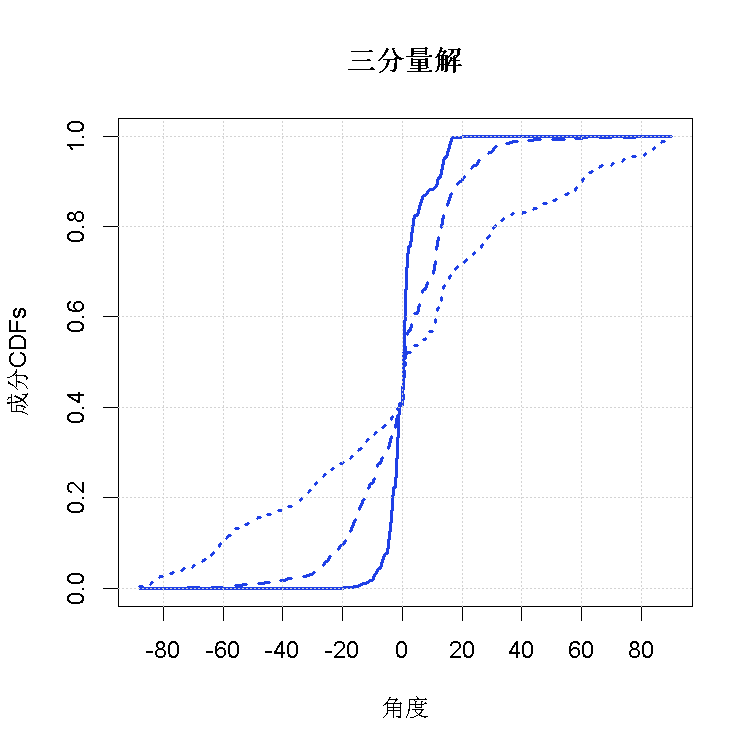图3(a)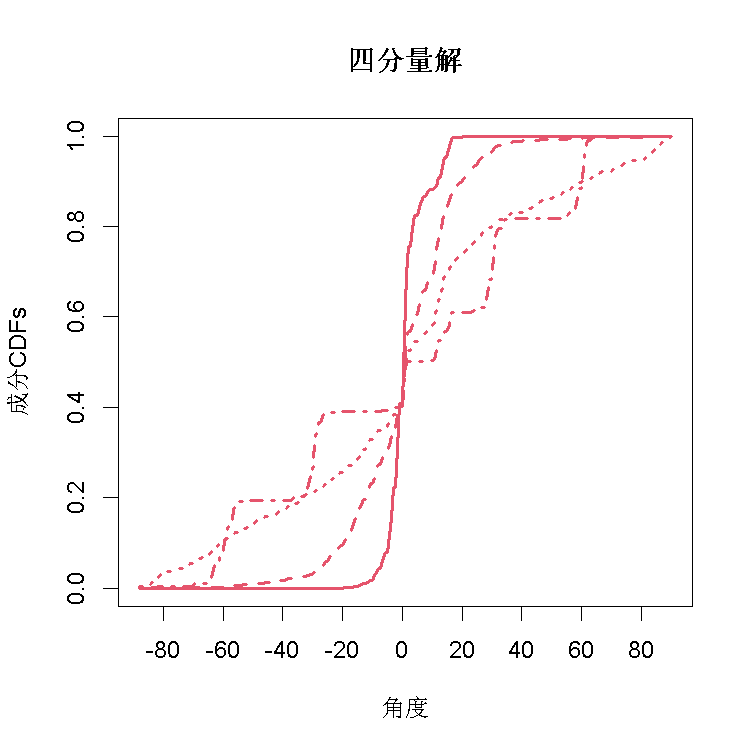图3(b)

同样可以用summary来总结EM输出。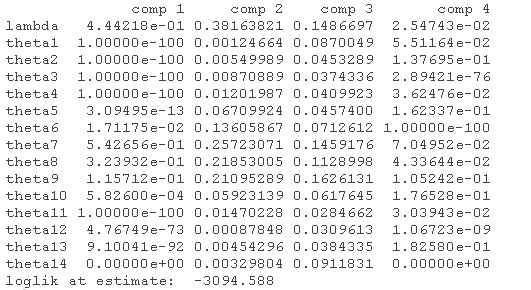单变量对称、位置偏移的半参数例子

在φ(-)相对于Lebesgue度量是绝对连续的额外假设下，Bordes等人(2007)提出了一种估计模型参数的随机算法，即(λ, µ, φ)。一个特例

R> plot(wait1, which = 2 )
R> wait2 <-EM(waiting)
R> plot(wait2, lty = 2)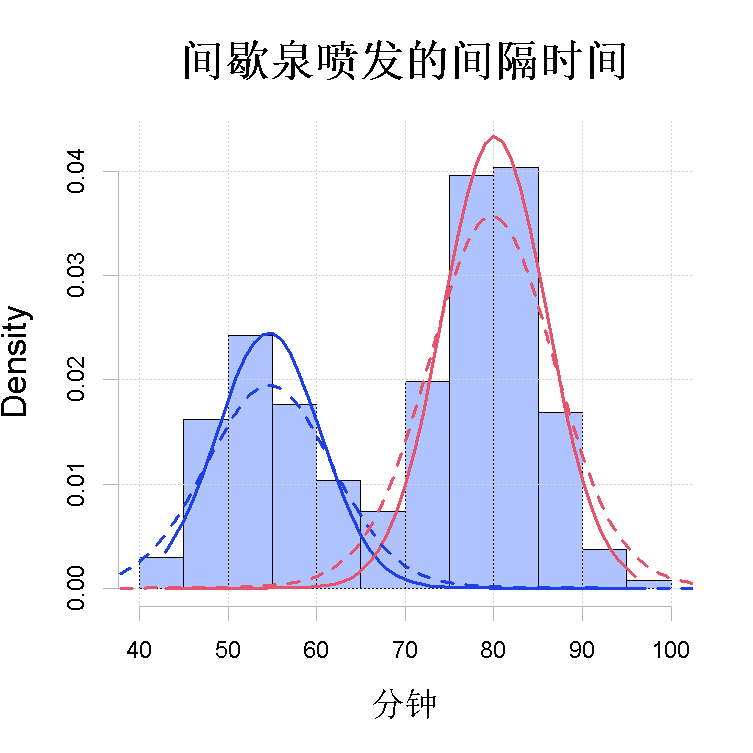图4(a)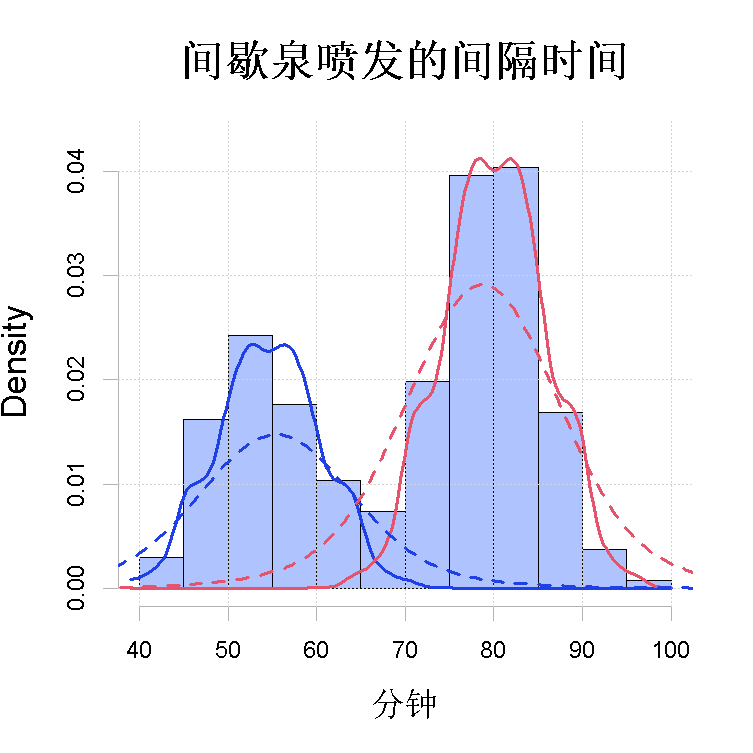图4(b)

因为半参数版本依赖于核密度估计步骤（8），所以有必要为这个步骤选择一个带宽。默认情况下，使用"Silverman的经验法则"（Silverman 1986）应用于整个数据集。

R> bw.nrd0(wait)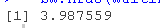但带宽的选择会产生很大的不同，如图4(b)所示。

> wait2a <- EM(wait, bw = 1)
> plot(wait2a
> plot(wait2b

我们发现，在带宽接近2的情况下，半参数解看起来非常接近图2的正态混合分布解。进一步降低带宽会导致图4(b)中的实线所表现出的 "凹凸不平"。另一方面，在带宽为8的情况下，半参数解效果很差，因为算法试图使每个成分看起来与整个混合分布相似。​

最受欢迎的见解

1.R语言k-Shape算法股票价格时间序列聚类

2.R语言中不同类型的聚类方法比较

3.R语言对用电负荷时间序列数据进行K-medoids聚类建模和GAM回归

4.r语言鸢尾花iris数据集的层次聚类

5.Python Monte Carlo K-Means聚类实战

6.用R进行网站评论文本挖掘聚类

7.用于NLP的Python：使用Keras的多标签文本LSTM神经网络

8.R语言对MNIST数据集分析 探索手写数字分类数据

9.R语言基于Keras的小数据集深度学习图像分类

展开全文R语言 EM算法 聚类
• emTutorial 教程可帮助教授EM算法背后的基本原理 设置 pip install -r requirements.txt 接触Python
• 对数的matlab代码GFMM 概述 此代码提供了研究论文的实现： ...生成模型 完全无人监管 扩展到大数据 执照 Copyright (C) This program is free software: you can redistribute it and/or modify it under the te
• 原创定制代写开发r/python/spss/ atlab/WEKA/sas/sql/C++/stata/eviews/Co puter science 原创定制代写开发r/python/spss/ atlab/WEKA/sas/sql/C++/stata/eviews/Co puter science assign ent 代写/代做Project/数据...
•研究论文
• 最近，针对有限混合体模型，特别是高斯混合模型，一种自动模型选择学习机制逐步发展成熟起来。这种新的机制能够在学习参数的过程中自动地完成模型选择，为数据的建模与分析提供了一种新的思路与途径。本文将对于高斯...
• 一个高斯混合模型是一个概率模型，它假设所有的数据点是从有限未知参数的高斯分布的混合生成的。可以将混合模型当作泛化的k均值聚类，以融合关于数据协方差和潜在高斯中心的信息。 高斯混合 GaussianMixture对象实现...
高斯混合模型
sklearn.mixture是一个能够学习高斯混合模型、抽样高斯模型和从数据中估计模型的包。同样，也提供了帮助决定正确组件数量的方法。
一个高斯混合模型是一个概率模型，它假设所有的数据点是从有限未知参数的高斯分布的混合生成的。可以将混合模型当作泛化的k均值聚类，以融合关于数据协方差和潜在高斯中心的信息。
高斯混合
GaussianMixture对象实现了expection-maximization算法来拟合高斯混合模型。它也能够得到多元模型的置信椭圆，计算贝叶斯信息准则来确定数据中聚集类别的数量。GaussianMixture.fit方法从训练数据中学习一个高斯混合模型，GaussianMixture.predict能够分配给每个样本最大可能属于的高斯分布。
GaussianMixture提供了不同的选项来限制不同类别估计的方差，包括，spherical、diagonal、tied或full方差。
# coding: utf-8
# GMM covariances

import matplotlib as mpl
import matplotlib.pyplot as plt

import numpy as np

from sklearn import datasets
from sklearn.mixture import GaussianMixture
from sklearn.model_selection import StratifiedKFold

colors = ['navy', 'turquoise', 'darkorange']

def make_ellipses(gmm, ax):
for n, color in enumerate(colors):
if gmm.covariance_type == 'full':
covariances = gmm.covariances_[n][:2, :2]
elif gmm.covariance_type == 'tied':
covariances = gmm.covariances_[:2, :2]
elif gmm.covariance_type == 'diag':
covariances = np.diag(gmm.covariances_[n][:2])
elif gmm.covariance_type == 'spherical':
covariances = np.eye(gmm.means_.shape) * gmm.covariances_[n]

v, w = np.linalg.eigh(covariances)
u = w / np.linalg.norm(w)
angle = np.arctan2(u, u)
angle = 180 * angle / np.pi
v = 2. * np.sqrt(2.) * np.sqrt(v)
ell = mpl.patches.Ellipse(gmm.means_[n, :2], v, v,
180+angle, color=color)
ell.set_clip_box(ax.bbox)
ell.set_alpha(0.5)
ax.add_artist(ell)
ax.set_aspect('equal', 'datalim')

iris = datasets.load_iris()

skf = StratifiedKFold(n_splits=4)

train_index, test_index = next(iter(skf.split(iris.data, iris.target)))

X_train = iris.data[train_index]
y_train = iris.target[train_index]
X_test = iris.data[test_index]
y_test = iris.target[test_index]

n_classes = len(np.unique(y_train))

estimators = {cov_type: GaussianMixture(n_components=n_classes, covariance_type=cov_type, max_iter=20, random_state=0)
for cov_type in ['spherical', 'diag', 'tied', 'full']}

n_estimators = len(estimators)

plt.figure(figsize=(3 * n_estimators // 2, 6))

plt.subplots_adjust(bottom=0.01, top=0.95, hspace=0.15, wspace=0.05, left=0.01, right=0.99)

for index, (name, estimator) in enumerate(estimators.items()):
estimator.means_init = np.array([X_train[y_train == i].mean(axis=0)
for i in range(n_classes)])

estimator.fit(X_train)

h = plt.subplot(2, n_estimators//2, index + 1)
make_ellipses(estimator, h)

for n, color in enumerate(colors):
data = iris.data[iris.target == n]
plt.scatter(data[:, 0], data[:, 1], s=0.8, color=color, label=iris.target_names[n])

for n, color in enumerate(colors):
data = X_test[y_test == n]
plt.scatter(data[:, 0], data[:, 1], marker='x', color=color)

y_train_pred = estimator.predict(X_train)
train_accuracy = np.mean(y_train_pred.ravel() == y_train.ravel()) * 100
plt.text(0.05, 0.9, 'Train accuracy: %.1f' % train_accuracy,
transform=h.transAxes)

y_test_pred = estimator.predict(X_test)
test_accuracy = np.mean(y_test_pred.ravel() == y_test.ravel()) * 100
plt.text(0.05, 0.8, 'Test accuracy: %.1f' % test_accuracy,
transform=h.transAxes)

plt.xticks(())
plt.yticks(())
plt.title(name)

plt.legend(scatterpoints=1, loc='lower right', prop=dict(size=12))

plt.show()

变分贝叶斯高斯混合
BayesianGaussianMixture对象实现了一系列考虑不同推断算法的高斯混合模型。
估计算法：变分推断
变分推断(Variational Inference)是最大期望的扩展，它最大化模型证据的下界，而不是数据似然。其背后的原理与最大期望方法相同。但是变分推断方法通过集成先验分布的信息添加正则项。这可以避免在最大期望中经常发生的奇异性，但会引入偏差到模型中。
BayesianGaussianMixture类提供了两类权重的先验：使用Dirichlet分布的有限混合模型和使用Dirichlet过程的无限混合模型。
# coding: utf-8
# Concentration Prior Type Analysis of Variation Bayesian Gaussian Mixture

import numpy as np

from sklearn.mixture import BayesianGaussianMixture

random_state, n_components, n_features = 2, 3, 2

covars = np.array([[[.7, .0], [.0, .1]],
[[.5, .0], [.0, .1]],
[[.5, .0], [.0, .1]]])
samples = np.array([200, 500, 200])
means = np.array([[.0, -.70],
[.0, .0],
[.0, .70]])

estimators = [
("Finite mixture with a Dirichlet distribution\nprior and "
r"$\gamma_0=$", BayesianGaussianMixture(
weight_concentration_prior_type="dirichlet_distribution",
n_components=2 * n_components, reg_covar=0, init_params='random',
max_iter=1500, mean_precision_prior=.8,
random_state=random_state), [0.001, 1, 1000]),
("Infinite mixture with a Dirichlet process\n prior and" r"$\gamma_0=$",
BayesianGaussianMixture(
weight_concentration_prior_type="dirichlet_process",
n_components=2 * n_components, reg_covar=0, init_params='random',
max_iter=1500, mean_precision_prior=.8,
random_state=random_state), [1, 1000, 100000])]

rng = np.random.RandomState(random_state)
X = np.vstack([
rng.multivariate_normal(means[j], covars[j], samples[j])
for j in range(n_components)])
y = np.concatenate([np.full(samples[j], j, dtype=int)
for j in range(n_components)])

for (title, estimator, concentrations_prior) in estimators:

for k, concentration in enumerate(concentrations_prior):
estimator.weight_concentration_prior = concentration
estimator.fit(X)
print(estimator.weights_)



展开全文聚类 python 机器学习
• 针对有限混合模型中参数估计方法对先验假设存在过分依赖的问题，提出了一种非参数的Legendre正交多项式图像混合模型分割方法。首先，设计了一种基于Legendre正交多项式的图像非参数混合模型，并用最小均方差(MISE)...正交多项式 图像分割
• 调整后的有限因变量混合模型是正态分布的有限混合，在极限处具有密度质量的累积，并且生活质量的100％与下一个较小的效用值之间存在差距。 软件包“ aldvmm”使用Hernandez Alava和Wailoo（2015）提出的似然和期望...
• 在本文中，我们基于有限混合回归模型研究了来自异类总体的时间序列数据的回归问题。 我们提出了两个有限的混合时变回归模型来解决这个问题。 提出了一种用于模型变量选择的正则化方法，该方法将适当的惩罚函数和l2...
• 提出了基于自由界面模态综合法的实验模型有限元分析模型混合建模方法。数值计算表明,基于剩余动柔度的剩余惯性释放附着模态更容易通过实验获得,而对剩余质量和剩余刚度的近似处理并不影响模态综合的精度。
• 由于人体脑血管结构复杂,空间比例小,三维分割和重构十分困难,本文面向时飞磁共振血管造影(TOF MRA)数据提出了一种新的瑞利高斯有限混合模型来实现脑血管的自动提取和分割。首先,对已有的混合模型进行了分析;然后,...
• 一种基于距离惩罚的混合模型分量数估计算法，张大明，符茂胜，EM算法是对有限混合模型进行参数估计的通用算法，然而标准EM算法所需的混合分量数实际上往往是未知的。为解决这个问题，本文研究��
• 采用界面元一有限元的混合模型来模拟三维土一结构体系。并采用一致边界来模拟波向无穷远域地基的传播作用。然后编制了一个可用于进行三维土一结构非线性地震反应分析的程序，并用该程序对一框架结构进行了考虑土一...
• 一般有限数目的混合模型... 对于有限混合模型中数据（data x）的密度函数的形式： ,其中 G是一个涵盖了混合参数的一个离散分布，G 的右半部分delta(theta)是以theta为中心的一块（区域）。Theta可做如下理解：
    一般有限数目的混合模型，贝叶斯提供一种基于数据的既可以估计混合模型中多少个components的数量，又可以估计每个mixture components 的参数值的方法。
对于有限混合模型中数据（data x）的密度函数的形式：,其中G是一个涵盖了混合参数的一个离散分布，G 的右半部分delta(theta)是以theta为中心的一块（区域）。Theta可做如下理解：类似筷子被截成n段，每一段是一个delta(theta)，这一段的中心值是theta。
当DPMM被应用于有限数据训练时，先采用有限的components对数据建模，因为每一个数据元对应一个component，而一个component可以对应多个不同的数据元，类似多对一的映射。
建模完成后，我们现在需要反过来推理模型中components的数量以及构成components的参数。
在这里我们就需要用到贝叶斯参数分析方法。先假设G服从某先验分布，因为G 是一个离散的多元分布，所以大多数情况下，首先映入脑海的是DP（DIRICHLET PROCESS）.这也是为什么要叫做DP混合模型的原因之一。
参见下图，含有K个component的DP混合模型，筷子截断后的多段中心theta 服从一个先验的分布H（lambda），就是每一段的中心值对应分布中的某一个值，这里多个段，也可以理解为一个cluster；Pi是衡量delta(theta)的权值，是一个狄里克雷分布【注：狄里克雷过程是一个离散的，狄里克雷分布是连续的】；Z是隐藏指示变量（可参考GMM内变量的阐述），由Z引出每一个cluster里观察到X的值。N代表N个cluster，X1....Xn。图中，空心圆表示变量，阴影圆表示可观测量，圆角矩形表示参数或者基本分布（eg.alpha一般选择gamma分布），矩形框代表迭代循环，矩形框右下角的数字则代表迭代循环的次数。
注意图中，G
的表示方法和文中对G 的描述，因为G一般采用stick-breaking来理解，这里引入潜在的指示变量Z（latent indicator
variables）来表征取G中的某一个值，这个值的权值为Pi，用以衡量delta(theta)的大小。，，Alpha 是一个测量参数或者一个分布，类似正态分布里的方差；H是一个基础测量值，类似正态分布里的均值。【IMPORTANT :::lamda=alpha*H】.
若对参数空间G进行划分，分为A1，...An，那么有（G（A1），...，G（An））~~~（alpha H(A1),....alpha H(An)）.
中餐馆过程可以很形象的帮助理解这个过程(CHINESE RESTAURANT PROCESS)。餐馆里有无数张桌子，这无数张桌子在实际中是用不完的，一般称为 countable infinite，我们在用到的有限数目的多少张桌子，这些桌子上分别都坐了多少个客人，新来的客人根据某些情况，以某种概率坐在已有的桌子上，或是另开辟一张新桌子。桌子的数目就和文中开篇提到的components保持对应，顾客就和parameters保持对应。


展开全文• 本文使用有限混合模型（Adams（2016））的最新结果，表明这可能是足够的信息来识别潜在的真实教育水平，而无需附加测量误差。 教育的估计回报与 Ashenfelter 和 Rouse (1998) 中提出的 IV 估计值相同或低于该估计值...
• EM算法是一种非常流行的最大似然估计方法，它是在观测数据为不完整数据时求解最大似然估计的迭代算法，同时也是估计有限混合模型参数的有效算法。 但是，EM算法不能保证找到全局最优解，而且往往容易陷入局部最优解...
• BRMM 类实现了用于模拟和估计有限混合模型参数的算法。 混合模型通常用于聚类分析，即将数据分组。 该模型专为包含异常值和/或缺失值的数据而设计。 BRMM 对象将每个原型建模为具有特定组件参数的重尾分布。 根据...matlab
• BRRMM 类实现了用于模拟和估计有限混合模型参数的算法。 回归混合模型通常用于聚类分析，即将输入-输出数据对分组。 该模型专为包含异常值的输出数据而设计。 BRRMM 对象将每个原型建模为具有特定于组件的参数的重...matlab
• 原文链接：... 泊松模型 proc fmm data = tmp1 tech = trureg; model majordrg = age acadmos minordrg logspend / dist = truncpoisson; probmodel age acadmos minordrg logspend;...
• SNOB是有限混合模型的Matlab实现。 Snob使用最小消息长度标准来估计混合模型的结构（即子种群的数量；哪个样本属于哪个子种群）并估计所有混合模型参数。 SNOB允许用户指定所需的子种群数量，但是，如果未指定，则...
• 为解决有限混合分布模型的聚类分析中分量密度函数选择问题，通过研究广义多元分析理论，提出了基于椭球等高分布混合模型的聚类算法．首先，利用与正态分布有许多相似性质的椭球等高分布族来构造混合分布模型，并引入...
• 原文链接：http://tecdat.cn/?p=6145... 泊松模型 proc fmm data = tmp1 tech = trureg; model majordrg = age acadmos minordrg logspend / dist = truncpoisson; probmodel age acadmos minord...
• 高斯混合模型是一种概率模型，它假设所有数据点都是从有限数量的高斯分布的混合参数中生成的。 1.高斯混合模型概念 实际上，可以将混合模型视为对 k-means聚类算法的扩展，它包含了数据的协方差结构以及隐高斯模型...大数据
• 本文给出了有限程排它过程与选举模型的混合模型遍历性的二个判别准则，从而推广和改进了已有的相应结果．...